# RBSE Maths Class 10 Chapter 3: Polynomials Important Questions and Solutions

RBSE Class 10 Maths Chapter 3 – Polynomials Important questions and solutions are given here. All these questions have detailed explanations, which help the students to understand easily. The RBSE Class 10 important questions and solutions, provided at BYJU’S, helps students to improve their problem-solving skills.

Chapter 3 of RBSE Class 10 Polynomials contains different concepts such as finding the zeroes of the polynomial, sum and product of zeroes also verifying the relationship between zeroes and coefficients of the given polynomial. Besides, there are some questions on finding all the zeroes of the polynomial using division method when some of them were given. This chapter also has quadratic equations, for which we need to find the factors with the help of different methods.

### RBSE Maths Chapter 3: Exercise 3.1 Textbook Important Questions and Solutions

Question 1: Find the zeroes of the quadratic polynomial 6x2 – x – 2 and verify the relationship between the zeroes and the coefficients.

Solution:

6x2 – x – 2

= 6x2 – 4x + 3x – 2

= 2x(3x – 2) + 1(3x – 2)

= (3x – 2)(2x + 1)

To find the zeroes, equate the given polynomial to 0.

3x – 2 = 0 ⇒ x = 2/3

2x + 1 = 0 ⇒ x = -1/2

Therefore, zeroes of the polynomial are 2/3 and -1/2.

Sum of zeroes = 2/3 + (-1/2) = 2/3 – 1/2 = (4 – 3)/6 = 1/6

Product of zeroes = (2/3) × (-1/2) = -1/3

Now comparing the given polynomial with the standard form ax2 + bx + c,

a = 6, b = -1, c = -2

Sum of zeroes = -b/a = -(-1)/6 = 1/6

Product of zeroes = c/a = -2/6 = -1/3

Hence, the relation between the zeroes and coefficients is verified.

Question 2: Find the zeroes of the quadratic polynomial x2 – (√3 + 1)x + √3 and verify the relationship between the zeroes and the coefficients.

Solution:

Given polynomial is:

x2 – (√3 + 1) x + √3

= x2 – √3x – x + √3

= x (x – √3) – 1(x – √3)

= (x – √3)(x – 1)

To find the zeroes, equate the given polynomial to 0.

x – √3 = 0 ⇒ x = √3

x – 1 = 0 ⇒ x = 1

Therefore, zeroes of the polynomial are √3 and 1.

Sum of zeroes = √3 + 1

Product of zeroes = (√3) (1) = √3

Now comparing the given polynomial with the standard form ax2 + bx + c,

a = 1, b = -(√3 + 1), c = √3

Sum of zeroes = -b/a = -(√3 + 1)/1 = √3 + 1

Product of zeroes = c/a = √3/1 = √3

Hence, the relation between the zeroes and coefficients is verified.

Question 3: Find a quadratic polynomial, whose sum and product of the zeroes is -3 and 2, respectively.

Solution:

Let α and β be the zeroes of the polynomial.

According to the given,

Sum of zeroes = α + β = -3

Product of zeroes = αβ = 2

If α and β are zeroes of any quadratic polynomial, then the quadratic polynomial is k[x2 – (α+β)x + αβ]

= k[x2 – (-3)x + 2]

= k(x2 + 3x + 2)

Where k is the proportionality constant.

Therefore, the required polynomial is x2 + 3x + 2.

Question 4: If the sum of squares of zeroes of quadratic polynomial f(x) = x2 – 8x + k is 40, then find the value of k.

Solution:

Given polynomial is:

x2 – 8x + k

Comparing with the standard form,

a = 1, b = -8, c = k

Let α and β are zeros of the polynomial.

Sum of zeroes = α + β = -b/a = -(-8)/1 = 8

Product of zeroes = αβ = c/a = k/1 = k

Now,

(α + β)2 = 82

α2 + β2 + 2αβ = 64

α2 + β2 + 2k = 64

40 + 2k = 64 (given sum of squares of zeroes is 40)

2k = 64 – 40

2k = 24

k = 24/2 = 12

Therefore, the value of k is 12.

### RBSE Maths Chapter 3: Exercise 3.2 Textbook Important Questions and Solutions

Question 5: Using division algorithm, divide f(x) by g(x) and find quotient and remainder.

f(x) = 3x3 + x2 + 2x + 5, g(x) = 1 + 2x + x2

Solution:

Given,

f(x) = 3x3 + x2 + 2x + 5, g(x) = 1 + 2x + x2

Using division algorithm,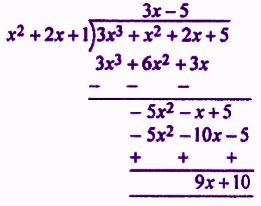Quotient = 3x – 5

Remainder = 9x + 10

Question 6: Using division algorithm, divide f(x) by g(x) and find quotient and remainder.

f(x) = x3 – 6x2 + 11x – 6, g(x) = x + 2

Solution:

Given,

f(x) = x3 – 6x2 + 11x – 6, g(x) = x + 2

Using division algorithm,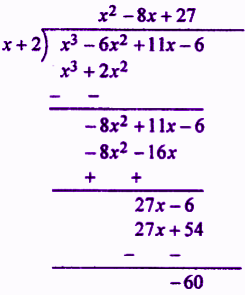Quotient = x2 – 8x + 27

Remainder = -60

Question 7: Using division algorithm, divide f(x) by g(x) and find quotient and remainder.

f(x) = 9x4 – 4x2 + 4, g(x) = 3x2 + x – 1

Solution:

Given,

f(x) = 9x4 – 4x2 + 4, g(x) = 3x2 + x – 1

Using division algorithm,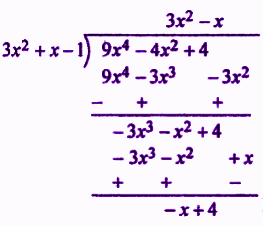Quotient = 3x2 – x

Remainder = -x + 4

Question 8: The zeroes of the polynomial f(x) = 2x4 – 3x3 – 3x2 + 6x – 2 are √2 and -√2. Find all other zeroes.

Solution:

Given polynomial is:

f(x) = 2x4 – 3x3 – 3x2 + 6x – 2

Two zeros of polynomial f(x) are √2 and -√2

(x – √2)(x + √2) = x2 – 2, will be a factor of f(x).

Dividing polynomial f(x) by x2 – 2,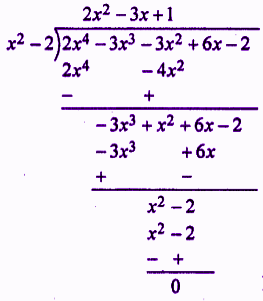Thus, f(x) = (x2 – 2)(2x2 – 3x + 1)

= (x2 – 2)(2x2 – 2x – x + 1)

= (x2 – 2) [2x(x – 1) – 1(x – 1)]

= (x2 – 2)(2x – 1)(x – 1)

To find the zeroes, take f(x) = 0.

2x – 1 = 0 ⇒ x = 1/2

x – 1 = 0 ⇒ x = 1

Therefore, all the zeroes of the given polynomial are √2, -√2, 1/2 and 1.

Question 9: The zeroes of the polynomial f(x) = x4 – 6x3 – 26x2 + 138x – 35 are 2 ± √3. Find all other zeroes.

Solution:

Given polynomial is:

f(x) = x4 – 6x3 – 26x2 + 138x – 35

Two zeros of the polynomial f(x) are 2 + √3 and 2 – √3.

Thus, [x – (2 + √3)][x – (2 – √3)] = [(x – 2) – √3][(x – 2) + √3]

= (x – 2)2 – (√3)2

= x2 – 4x + 4 – 3

= x2 – 4x + 1

x4 – 4x + 1 is the factor of f(x).

Dividing f(x) by x2 – 4x + 1,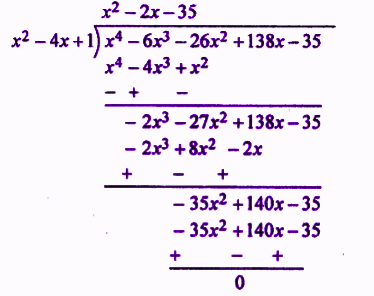f(x) = x4 – 6x3 – 26x2 + 138x – 35 = (x2 – 4x + 1)(x2 – 2x – 35)

= (x2 – 4x + 1)(x2 – 7x + 5x – 35)

= (x2 – 4x + 1) [x(x – 7) + 5(x – 7)]

= (x2 – 4x + 1)(x + 5)(x – 7)

Other zeroes of the polynomial are:

x + 5 = 0 ⇒ x = -5

x – 7 = 0 ⇒ x = 7

Therefore, all the zeroes of the given polynomial are 2 ± √3, -5 and 7.

Question 10: The zero of the polynomial f(x) = x3 + 13x2 + 32x + 20 is -2. Find all other zeroes.

Solution:

Given polynomial is:

f(x) = x3 + 13x2 + 32x + 20

One zero is -2.

(x + 2) is the factor of f(x).

Dividing f(x) by x + 2,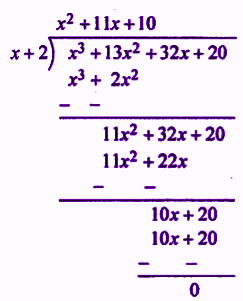f(x) = x3 + 13x2 + 32x + 20 = (x + 2)(x2 + 11x + 10)

= (x + 2)(x2 + 10x + x + 10)

= (x + 2) [x(x + 10) + 1(x + 10)]

= (x + 2)(x + 1)(x + 10)

Other zeroes of the polynomial are:

x + 1 = 0 ⇒ x = -1

x + 10 = 0 ⇒ x = -10

Therefore, all the zeroes of the given polynomial are -2, -1 and -10.

### RBSE Maths Chapter 3: Exercise 3.3 Textbook Important Questions and Solutions

Question 11: Check whether x(x + 1) + 8 = (x + 2)(x – 2) is quadratic.

Solution:

x(x + 1) + 8 = (x + 2)(x – 2)

x2 + x + 8 = x2 + 2x – 2x – 4

x2 + x + 8 = x2 – 4

x2 + x + 8 – x2 + 4 = 0

x + 12 = 0

The degree of the equation is 1.

Therefore, the given equation is not a quadratic equation.

Question 12: Check whether x + 1/x + x2 = 0, x ≠ 0 is quadratic.

Solution:

x + 1/x + x2 = 0

⇒ (x2 + 1 + x3)/x = 0

⇒ x3 + x2 + 1 = 0

The degree of the equation is 3.

Therefore, the given equation is not a quadratic equation.

Question 13: Check whether (x + 2)3 = x3 – 4 is quadratic.

Solution:

(x + 2)3 = x3 – 4

x3 + 3(x)(2)(x + 2) + (2)3 = x3 – 4

x3 + 6x(x + 2) + 8 = x3 – 4

x3 + 6x2 + 12x + 8 – x3 + 4 = 0

6x2 + 12x + 12 = 0

6(x2 + 2x + 2) = 0

x2 + 2x + 2 = 0

The degree of the equation is 2.

Hence, the given equation is a quadratic equation.

Question 14: Solve the following equation by factorisation method.

2x2 – 5x + 3 = 0

Solution:

Given,

2x2 – 5x + 3 = 0

2x2 – 2x – 3x + 3 = 0

2x(x – 1) – 3(x – 1) = 0

(2x – 3)(x – 1) = 0

2x – 3 = 0 ⇒ x = 3/2

x – 1 = 0 ⇒ x = 1

Therefore, the roots of the given quadratic equation are 1 and 3/2.

Question 15: Solve the following equation by factorisation method.

√3x2 + 10x + 7√3 = 0

Solution:

√3x2 + 10x + 7√3 = 0

√3x2 + 3x + 7x + 7√3 = 0

√3x(x + √3) + 7(x + √3) = 0

(√3x + 7)(x + √3) = 0

√3x + 7 = 0, x + √3 = 0

x = -7/√3, x = -√3

Therefore, the roots of the given equation are -7/√3 and -√3.

Question 16: Solve the following equation by factorisation method.

1/(x – 2) + 2/(x – 1) = 6/x where x ≠ 1, 2

Solution:

Given equation is:

1/(x – 2) + 2/(x – 1) = 6/x

[(x – 1) + 2(x – 2)]/ (x – 1)(x – 2) = 6/x

(x – 1 + 2x – 4)/(x2 – x – 2x + 2) = 6/x

(3x – 5)/(x2 – 3x + 2) = 6/x

By cross multiplying LHS and RHS,

6(x2 – 3x + 2) = x(3x – 5)

6x2 – 18x + 12 = 3x2 – 5x

6x2 – 18x + 12 – 3x2 + 5x = 0

3x2 – 13x + 12 = 0

3x2 – 9x – 4x + 12 = 0

3x(x – 3) – 4(x – 3) = 0

(3x – 4)(x – 3) = 0

3x – 4 = 0, x – 3 = 0

x = 4/3, x = 3

Therefore, the roots of the given equation are 4/3 and 3.

Question 17: Solve the following equation by factorisation method.

3x2 – 2√6x + 2 = 0

Solution:

3x2 – 2√6x + 2 = 0

(√3x)2 – 2(√3x)(√2) + (√2)2 = 0

(√3x – √2)2 = 0

(√3x – √2)(√3x – √2) = 0

√3x – √2 = 0, √3x – √2 = 0

x = √(2/3), x =√(2/3)

Therefore, the roots of the given quadratic equation are √(2/3) and √(2/3).

Question 18: Solve the following equation by factorisation method.

(x + 3)/(x + 2) = (3x – 7)/(2x – 3)

Solution:

(x + 3)/(x + 2) = (3x – 7)/(2x – 3)

By cross multiplying LHS and RHS,

(x + 3)(2x – 3) = (x + 2)(3x – 7)

2x2 – 3x + 6x – 9 = 3x2 – 7x + 6x – 14

2x2 + 3x – 9 = 3x2 -x – 14

3x2 – x – 14 – 2x2 – 3x + 9 = 0

x2 – 4x – 5 = 0

x2 – 5x + x – 5 = 0

x(x – 5) + 1(x – 5) = 0

(x – 5)(x + 1) = 0

x – 5 = 0, x + 1 = 0

x = 5, x = -1

Therefore, the roots of the given equation are 5 and -1.

Question 19: Solve the following equation by factorisation method.

4x2 – 4a2x + (a4 – b4) = 0

Solution:

4x2 – 4a2x + (a4 – b4) = 0

4x2 -[2(a2 + b2) + (a2 – b2)x + (a2 + b2)(a2 – b2) = 0

4x2 – 2(a2 + b2)x – 2(a2 – b2)x + (a2 + b2)(a2 – b2) = 0

2x[2x – (a2 + b2)] – (a2 – b2)[2x – (a2 + b2)] [2x – (a2 – b2)] [2x – (a2 + b2)] = 0

2x – (a2 – b2) = 0, 2x – (a2 + b2) = 0

x = (a2 – b2)/2, x = (a2 + b2)/2

Therefore, the roots of the given equation are (a2 – b2)/2 and (a2 + b2)/2.

Question 20: Solve the following equation by factorisation method.

abx2 + (b2 – ac)x – bc = 0

Solution:

abx2 + (b2 – ac)x – bc = 0

abx2 + b2x – acx – bc = 0

bx(ax + b) – c(ax + b) = 0

(bx – c)(ax + b) = 0

bx – c = 0, ax + b = 0

x = c/b, x = -b/a

Therefore, the roots of the given equation are c/b and -b/a.

### RBSE Maths Chapter 3: Exercise 3.4 Textbook Important Questions and Solutions

Question 21: Solve the following quadratic equation by the method of perfect square.

3x2 – 5x + 2 = 0

Solution:

3x2 – 5x + 2 = 0

Dividing by 3,

x2 – (5/3)x + (2/3) = 0

x2 – (5/3)x = -2/3

Adding the square of half the coefficient of x on both sides,

x2 – 2(5/6)x + (5/6)2 = 2(2/3) + (5/6)2

(x – 5/6)2 = (25/36) – (2/3)

(x – 5/6)2 = (25 – 24)/36

(x – 5/6)2 = 1/36

x – 5/6 = ± 1/6

Now,

x – 5/6 = 1/6, x – 5/6 = -1/6

x = 5/6 + 1/6, x = 5/6 – 1/6

x = 6/6, x = 4/6

x = 1, x = 2/3

Therefore, roots of the given equation are 1 and 2/3.

Question 22: Solve the following quadratic equation by the method of perfect square.

4x2 + 3x + 5 = 0

Solution:

4x2 + 3x + 5 = 0

Dividing by 4,

x2 + (3/4)x + (5/4) = 0

x2 + (3/4)x = -5/4

Adding half the square of coefficient of x on both sides,

x2 + (3/4)x + (3/8)2 = -(5/4) + (3/8)2

x2 + 2(3/8)x + (3/8)2 = (9/64) – (5/4)

(x + 3/8)2 = (9 – 80)/64

(x + 3/8)2 = -71/64

Here, RHS is negative.

Therefore, roots are not real.

Question 23: Find the roots of the following quadratic equation, if they exist, using the quadratic formula of Shridhar Acharya.

x + 1/x = 3, x ≠ 0

Solution:

x + 1/x = 3, x ≠ 0

(x2 + 1)/x = 3

x2 + 1 = 3x

x2 – 3x + 1 = 0

Comparing with the general form ax2 + bx + c = 0,

a = 1, b = -3 and c = 1

Discriminant = b2 – 4ac

= (-3)2 – 4(1)(1)

= 9 – 4

= 5 > 0

Thus, the roots are real.

Using Shridhar Acharya formula,

x = [-b ± √(b2 – 4ac)]/ 2a

x = [-(-3) ± √5]/ 2(1)

x = (3 ± √5)/2

Therefore, the roots of the given equation are (3 + √5)/2 and (3 – √5)/2.

Question 24: Find the roots of the following quadratic equation, if they exist, using the quadratic formula of Shridhar Acharya.

x2 + 4x + 5 = 0

Solution:

x2 + 4x + 5 = 0

Comparing with the general form ax2 + bx + c = 0,

a = 1, b = 4, c = 5

Discriminant = b2 – 4ac

= (4)2 – 4(1)(5)

= 16 – 20

= – 4 < 0

Therefore, the roots of the quadratic equation are not real.

Question 25: Find two consecutive positive odd integers, sum of whose squares is 290.

Solution:

Let x and x + 2 be the two consecutive odd positive integers.

According to the given,

(x)2 + (x + 2)2 = 290

x2 + x2 + 4x + 4 = 290

2x2 + 4x + 4 = 290

2x2 + 4x – 286 = 0

x2 + 2x – 143 = 0

x2 + 13x – 11x – 143 = 0

x(x + 13) – 11(x + 13) = 0

(x + 13) (x – 11) = 0

x – 11 = 0, x + 13 = 0

x = 11, x = -13

Since x is a positive integer, we ignore -13.

Therefore, x = 11

x + 2 = 11 + 2 = 13

Hence, two consecutive positive odd integers are 11, 13.

Question 26: The difference of square of two numbers is 45 and square of smaller number is four times the larger number. Find the two numbers.

Solution:

Let x and y be the two numbers and x > y.

According to the given,

x2 – y2 = 45 ….(i)

y2 = 4x ….(ii)

From (i) and (ii),

x2 – 4x = 45

x2 – 4x – 45 = 0

x2 – 9x + 5x – 45 = 0

x(x – 9) + 5 (x – 9) = 0

(x – 9) (x + 5) = 0

x – 9 = 0

x = 9

Therefore, the larger number = x = 9

Square of smaller number = y2 = 4 × 9 = 36

y = ±√36 = ±6

Hence, the two numbers are 9, 6 or 9, -6.

Question 27: Divide 16 into two equal parts such that 2 times the square of the larger part is 164 more than the square of the smaller part.

Solution:

Let x be the larger part and the smaller part be 16 – x.

According to question,

2x2 = (16 – x)2 + 164

2x2 – (16 – x)2 – 164 = 0

2x2 – (256 – 32x + x2) – 164 = 0

2x2 – 256 + 32x – x2 – 164 = 0

x2 + 32x – 420 = 0

x2 + 42x – 10x – 420 = 0

x(x + 42) – 10(x + 42) = 0

(x + 42) (x – 10) = 0

x = -42 or x = 10

x = 10 (since x = -42 not possible)

Therefore, the larger number = x = 10

Smaller number = 16- x = 16 – 10 = 6

Hence, the two numbers are 10 and 6.

### RBSE Maths Chapter 3: Exercise 3.5 Textbook Important Questions and Solutions

Question 28: Find the nature of roots of the following quadratic equation.

2x2 – 4x + 3 = 0

Solution:

2x2 – 4x + 3 = 0

Comparing with the standard form ax2 + bx + c = 0,

a = 2, b = – 4 and c = 3

Discriminant(D) = b2 – 4ac

= (-4)2 – 4 × 2 × 3

= 16 – 24

= -8 < 0

Therefore, the given equation has no real roots.

Question 29: Find the nature of roots of the following quadratic equation.

2x2 + x – 1 = 0

Solution:

2x2 + x – 1 = 0

Comparing with the standard form ax2 + bx + c = 0,

a = 2, b = 1 and c = -1

Discriminant (D) = b2 – 4ac

= (1)2 – 4 (2)(-1)

= 1 + 8

= 9 > 0

Therefore, the roots of the given equation are real and distinct.

Question 30: Find the nature of roots of the following quadratic equation.

x2 – 4x + 4 = 0

Solution:

x2 – 4x + 4 = 0

Comparing with the standard form ax2 + bx + c = 0,

a = 1, b = -4 and c = 4

Discriminant (D) = b2 – 4ac

= (-4)2 – 4 (1)(4)

= 16 – 16

= 0

Therefore, the roots of the given equation are real and equal.

Question 31: Find the value of k in the following quadratic equation for which roots are real and equal.

x2 – 2(k + 1)x + k2 = 0

Solution:

x2 – 2(k + 1)x + k2 = 0

Comparing with the standard form ax2 + bc + c = 0,

a = 1, b = -2(k + 1) and c = k2

Given that, the quadratic equation has real and equal roots.

That means, discriminant (D) = 0

b2 – 4ac = 0

[-2(k + 1)]2 – 4 × 1 × k2 = 0

4(k2 + 2k + 1) – 4k2 = 0

4k2 + 8k + 4 – 4k2 = 0

8k + 4 = 0

8k = -4

k = -4/8

k = -1/2

Question 32: Find the value of k for which the quadratic equation kx2 + 2x + 1 = 0 has real and distinct roots.

Solution:

kx2 + 2x + 1 = 0

Comparing with the standard form ax2 + bx + c = 0,

a = k, b = 2, c = 1

Given that, the roots of the given equation are real and distinct.

That means, discriminant (D) > 0

b2 – 4ac > 0

(2)2 – 4(k)(1) = 0

4 – 4k > 0

4(1 – k) > 0

1 – k > 0

1 > k

Or

k < 1

Therefore, k takes all the real numbers which are less than 1.

Question 33: Find the value of k so that equation x2 + 5kx + 16 = 0 has no real roots.

Solution:

x2 + 5kx + 16 = 0

Comparing with the standard form ax2 + bx + c = 0,

a = 1, b = 5k, c = 16

Given that, the given equation has no real roots.

That means, discriminant (D) < 0

b2 – 4ac < 0

(5k)2 – 4(1)(16) = 0

25k2 – 64 < 0

25k2 < 64

k2 < 64/25

k < ± 8/5

Therefore, the value of k lies between -8/5 and 8/5.

Question 34: If roots of the quadratic equation (b – c)x2 + (c – a)x + (a – b) = 0 are real and equal, then prove that 2b = a + c.

Solution:

Given quadratic equation is (b – c)x2 + (c – a)x + (a – b) = 0

Comparing with the standard quadratic equation ax2 + bx + c = 0,

a = (b – c), b = (c – a) and c = (a – b)

Given that, the roots are real and equal.

That means, discriminant (D) = 0

b2 – 4ac = 0

(c – a)2 – 4(b – c)(a – b) = 0

(c2 + a2 – 2ac) – 4(ab – ac – b2 + bc) = 0

c2 + a2 – 2ac – 4ab + 4ac + 4b2 – 4bc = 0

c2 + a2 + 2ac – 4ab – 4bc + 4b2 = 0

(a + c)2 – 4 b(a + c) + 4b2 = 0

(a + c)2 – 2 × (a + c) × 2b + (2b)2 = 0

[(a + c) – 2b]2 = 0

(a + c) – 2b = 0

a + c = 2b

Hence proved.

### RBSE Maths Chapter 3: Exercise 3.6 Textbook Important Questions and Solutions

Question 35: Find the LCM of the following expressions.

24x2yz and 27x4y2z2

Solution:

24x2yz = 2 × 2 × 2 × 3 × x2 × y × z = 23 × 3 × x2 × y × z

27x4y2z2 = 3 × 3 × 3 × x4 × y2 × z2 = 33 × x4 × y2 × z2

Therefore, LCM = 23 × 33 × x4 × y2 × z2

= 8 × 27 × x4y2z2

= 216x4y2z2

Question 36: Find the LCM of the following expressions.

x2 – 3x + 2 and x4 + x3 – 6x2

Solution:

x2 – 3x + 2 = x2 – 2x – x + 2

= x(x – 2) -1(x – 2)

= (x – 1)(x – 2)

x4 + x3 – 6x2 = x2(x2 + x – 6)

= x2(x2 + 3x – 2x – 6)

= x2[x(x + 3) – 2(x + 3)]

= x2(x – 2)(x + 3)

Therefore, LCM = x2(x – 1)(x – 2)(x + 3)

Question 37: Find the HCF of the following expressions.

a3b4, ab5, a2b8

Solution:

Given expressions are:

a3b4 = a3 × b4

ab5 = a × b5

a2b8 = a2 × b8

HCF is the product of least powers of common factors in the factorisation.

Therefore, HCF = a × b4 = ab4

Question 38: Find the HCF of the following expressions.

(x + 3)2(x – 2) and (x + 3)(x – 2)2

Solution:

Given expressions are:

(x + 3)2(x – 2) and (x + 3)(x – 2)2

HCF is the product of least powers of common factors in the factorisation.

Therefore, HCF = (x + 3)(x – 2)

Question 39: If u(x) = (x – 1)2 and v(x) = (x2 – 1), then verify LCM × HCF = u(x) × v(x)

Solution:

Given,

u(x) = (x – 1)2 and v(x) = (x2 – 1)

u(x) = (x – 1)2 = (x – 1)(x – 1)

v(x) = x2 – 1 = x2 – 12 = (x + 1)(x – 1)

LCM = (x – 1)2(x + 1)

HCF = (x – 1)

LCM × HCF = (x – 1)2(x + 1)(x – 1) = (x – 1)2(x2 – 1)

u(x) × v(x) = (x – 1)2(x2 – 1)

Therefore, LCM × HCF = u(x) × v(x)

Hence proved.

Question 40: The product of two expressions is (x – 7)(x2 + 8x + 12). If their highest common factor (HCF) is (x + 6), then find their least common multiple (LCM).

Solution:

Given,

Product of two expressions = (x – 7)(x2 + 8x + 12)

= (x – 7) [x2 + 6x + 2x + 12]

= (x – 7) [x(x + 6) + 2(x + 6)]

= (x – 7)(x + 2)(x + 6)

HCF = x + 6 (given)

We know that,

LCM × HCF = Product of two given expressions

LCM = Product of two expressions/HCF

= (x – 7)(x + 2)(x + 6)/(x + 6)

= (x – 7)(x + 2)

= x2 + 2x – 7x – 14

= x2 – 5x – 14

Therefore, the LCM is x2 – 5x – 14.

### RBSE Maths Chapter 3: Additional Important Questions and Solutions

Question 1: If one zero of polynomial f(x) = 5x2 + 13x + k is reciprocal to other zero, then value of k will be:

(a) 0

(b) 1/5

(c) 5

(d) 6

Solution:

Given polynomial is f(x) = 5x2 + 13x + k

Comparing with the standard form ax2 + bx + c,

a = 5, b = 13, c = k

Let α and 1/α be the two zeroes of the given polynomial.

Product of zeroes = α × (1/α) = c/a

1 = k/5

k = 5

Question 2: If 3 is a zero of polynomial 2x2 + x + k, then value of k will be:

(a) 12

(b) 21

(c) 24

(d) -21

Solution:

Let the given polynomial be p(x) = 2x2 + x + k

3 is the zero of a given polynomial.

Therefore, p(3) = 0

2(3)2 + 3 + k = 0

2(9) + 3 + k = 0

18 + 3 + k = 0

21 + k = 0

k = -21

Question 3: If α, β are zeroes of polynomial x2 – p(x + 1) – c such that (α + 1)(β + 1) = 0, then value of c will be:

(a) 0

(b) -1

(c) 1

(d) 2

Solution:

Given that, α, β are zeroes of the polynomial x2 – p(x + 1) – c.

x2 – p(x + 1) – c = x2 – px – p – c

= x2 – px – (p + c)

Comparing with the standard form,

a = 1, b = -p, c = -(p + c)

Sum of zeroes = α + β = -b/a = -(-p)/1 = p

Product of zeroes = αβ = c/a = -(p + c)/1 = -(p + c)

Now,

(α + 1)(β + 1) = 0 (given)

αβ + α + β + 1 = 0

-(p + c) + p + 1 = 0

-p – c + p + 1 = 0

1 – c = 0

c = 1

Question 4: Nature of roots of quadratic equation 4x2 – 12x – 9 = 0 is

(a) real and equal

(b) real and distinct

(c) imaginary and equal

(d) imaginary and distinct

Solution:

4x2 – 12x – 9 = 0

Comparing with the standard form,

a = 4, b = -12, c = -9

Discriminant (D) = b2 – 4ac

= (-12)2 – 4 × 4 × (-9)

= 144 + 144

= 288 > 0

Therefore, the roots are real and distinct.

Question 5: Write Shridhar Acharya’s formula to find roots of quadratic equation ax2 + bx + c = 0.

Solution:

Shridhar Acharya formula to find the roots of the quadratic equation is:

x = [-b ± √(b2 – 4ac)]/ 2a

Where, a = coefficient of x2

b = coefficient of x

c = constant term

Question 6: If α and β are zeroes of quadratic polynomial f(x) = x2 – px + q, then find the values of the following:

(i) α2 + β2 (ii) (1/α) + (1/β)

Solution:

Given quadratic polynomial is f(x) = x2 – px + q

Comparing f(x) with the standard form,

a = 1, b = -p, c = q

Sum of zeroes = α + β = -b/a = -(-p)/1 = p

Product of zeroes = αβ = c/a = q/1 = q

(i) α2 + β2 = (α + β)2 – 2αβ = p2 – 2q

(ii) (1/α) + (1/β) = (α + β)/αβ = p/q

Question 7: Divide x3 – 6x2 + 11x – 6 by x – 2 and verify the division algorithm.

Solution:

Let f(x) = x3 – 6x2 + 11x – 6 and g(x) = x – 2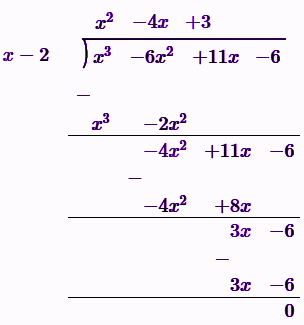Quotient = q(x) = x2 – 4x + 3

Remainder = 0

Using division algorithm,

f(x) = g(x) q(x) + Remainder

= (x – 2)(x2 – 4x + 3) + 0

= x3 – 4x2 + 3x – 2x2 + 8x – 6

= x3 – 6x2 + 11x – 6

Hence verified.